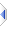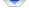# Multiple Assignment

Sometimes it can be convenient to make several assignments in one statement, for example
```    {a,b,c} = somefunc()
```
is (functionally) the same as
```    tmp = somefunc()
c = tmp
b = tmp
a = tmp
```
Alternatively you may want to initialise several items to the same thing, eg
```    {names,dates,sizes} @= {}
```
which is functionally the same as
```    sizes = {}
dates = {}
names = {}
```
Like everywhere else, instead of the plainer "=" you can use ":=", which is normally pronounced "becomes equal to", whereas "@=" is normally pronounced "all become equal to". (The comparison form of "=", "==", which is normally pronounced "is equal to", is not used anywhere else on this page.)

You can also nest (to any level) and combine the two types of multiple assignment:
```    {{a,b},{c,d}} = {{1,2},{3,4}}       -- (becomes equal to)
```
is functionally equivalent to
```    {c,d} = {3,4}
{a,b} = {1,2}
```
which in turn is functionally equivalent to
```    d = 4
c = 3
b = 2
a = 1
```
whereas
```    {{a,b},{c,d}} @= {{1,2},{3,4}}      -- (all become equal to)
```
is functionally equivalent to (any nested multiple assignments are always the plainer "="/":=")
```    {c,d} = {{1,2},{3,4}}
{a,b} = {{1,2},{3,4}}
```
which is the same as
```    d = {3,4}
c = {1,2}
b = {3,4}
a = {1,2}
```
Subscripts and slices are also permitted; if s is {1,2,3,4} then
```    {s,s[2..\$]} = {s[\$],s[1..\$-1]}
```
leaves s as {4,1,2,3}. It would require named temporary variables to achieve the same thing using multiple statements, whereas the above form uses unnamed temps, which, obviously enough, need not (and indeed cannot) be declared. However see performance notes below (multiple assignment is designed for comfort and convenience not speed).

As well as the syntax previously shown in Variables and Constants, you can also declare variables as part of (/mid-) multiple assignment, eg
```    {string name, integer id} = lookup()
```
however sub-types do /NOT/ propagate/carry-over-commas as you might expect, eg:
```    {a, string b, c} = lookup()
```
will terminate in error if b already exists, or if a /or c/ does not already exist. Use "string b, string c" instead, along with whatever you might need to do for a. While string {a, b, c} propagates the type, and declares three new variables of type string, that does /not/ happen for types inside the {}, except when the type immediately precedes an opening/nested '{'. Admittedly this is a simple practical choice/implementation detail (see pmain.e/GetMultiAssignSet()) that it may be possible to improve upon, but there are four competing use cases for that routine, hence the simplest solution won (being reset after every comma). Also, constructs such as string {a, integer b, c} are treated as nonsense and trigger an error, but obviously you can simply move the "string" inside the {}, in that particular case twice.

Use '{}' (or '?') to omit elements
```    {a,{},c} = {1,2,3}
```
is functionally the same as
```    c = 3
a = 1
```
and ditto for "{a,?,c} = {1,2,3}".

You can also omit everything:
```    {} = f()
```
which explicitly discards the result of the function call. Alternatively if you prefer `{?} = f()` has exactly the same effect (however `? = f()` is not legal syntax). Note that OpenEuphoria (imnsho wrongly) allows /implicit/ discarding of function results, which phix does not, whereas, cmiiw, OpenEuphoria does not support any of the subscripting, nesting, "@=", "{}=", ":=", or "==" forms of (multiple) assignment that phix does.

Like all powerful programming constructs, multiple assigment can be used to make things easier, but it can also be abused to make things much more difficult than they need to be.

## How NOT to use multiple assigment

You may be wondering why I appear to have written the equivalents "backwards". Any subscripts on the lhs are pushed onto opstack (in pmain.e) from left to right, hence the assignments are always performed from right to left to pick up any subscripts from the top of the stack, in the right order. (Actually, there is an exception to that rule, as explained in the technicalia section below.) Of course relying on such subtleties would constitute very bad practice, and in reality that is a fairly trivial implementation detail that could fairly easily be changed, if it ever proves necessary that is.

One case where this may trip you is:
```    {main,mainHwnd} = {create(Window,...),getHwnd(main)} -- error!
```
Obviously you could rewrite that statement the other way round, but as the saying goes, debugging is several times harder than writing code in the first place, therefore if you write tricky code you are, by definition, not smart enough to debug it.

Update: actually, you cannot write that "the other way round". Further illustrating the "not smart enough" point, I failed to spot that the rhs is constructed in its entireity before any assignments on the lhs, hence main would be unassigned whichever way round things were done [ie, as mentioned elsewhere, you should never try to both modify and reference the same variable in a single statement, in any programming language].

Another example is the slightly dodgy:
```    {s[idx],idx} = <something>
```
which is perhaps understandable, but may be "an accident waiting to happen". Clearly someone could read that and assume it is setting the old idx of s, before getting a new value of idx for something else later, but of course that would be the effect of {idx,s[idx]} = <something>. Not nice, really, either way round.

Certainly the lhs should never rely on something having "already happened" on the rhs, or otherwise attempt to modify something twice in a single statement, except for "static" subscripts. As an example to clarify that last point, if you try to make various substitutions in say "The [noun] [verb] the [object]", all in a single statement and possibly in any order, and further the lengths and positions all change several times mid-statement, expect problems! Do them one at a time, and figure out where things are, after, rather than before they have moved(!!), and it should all be plain sailing.
<aside>

OK, to further clarify that, consider this longhand version:
```            s = "The [noun] [verb] on the [object]"
--   123456789012345678901234567890123
--           -1-       -2-       -3-
s[26..33] = "mat"
s[12..17] = "sat"
s[5..10] = "cat"
```
which is fine, but you should really avoid this nastiness:
```            {s[26..33],s[12..17],s[5..10]} = {"mat","sat","cat"}
```
or this nasty mess:
```            {s[5..10],s[12..17],s[26..33]} = {"cat","sat","mat"}
```
I bet that intuitively, if told one of them does not work most people would assume the first works but the second does not, when in fact it is the other way round. Few people would (immediately) understand what I have done with the indexes to get (/force) these alternatives to work:
```            {s[20..27],s[9..14],s[5..10]} = {"mat","sat","cat"}
{s[20..27],s[5..10],s[12..17]} = {"mat","cat","sat"}
{s[9..14],s[23..30],s[5..10]} = {"sat","mat","cat"}
{s[9..14],s[5..10],s[26..33]} = {"sat","cat","mat"}
{s[5..10],s[23..30],s[12..17]} = {"cat","mat","sat"}
```
(Notice how "mat" goes to s[20..27], s[23..30] or s[26..33]) Of course in a real world program, we would not use fixed literal integers but the results from find or match, and dynamically apply adjustments to those results. Technically it can be made to work, but regardless, this sort of stuff is truly horrid, don’t do it! (except perhaps when participating in a code obfuscation contest)

</aside>
Another example occured when writing the rosettacode/Reduced_row_echelon_form entry. I tried to replace
```sequence temp
temp = M[i]
M[i] = M[r]
```
with
```        {M[i],M[r]} = {M[r],sq_div(M[i],M[i][lead])
```
It failed miserably when i=r, for the same "right to left" reasons (there is nothing wrong with the rhs per se, but the lhs overwrites in the wrong order). If it was my code to maintain, I would probably leave the top version in, but after some consideration I decided that a fixed version of the one-liner, with an appropriate comment, was probably the more useful thing in that particular instance.

The "{s,s[2..\$]} = {s[\$],s[1..\$-1]}" example already given is perhaps the most complex thing that can be considered "static" subscripts. No s[i] is updated twice and there is no confusion or dependency on any destination, though admittedly it relies on all "gathering" occuring before any update, which is a general rule for all forms of assignment anyway. (And I should quickly remind you, again, to check out the performance notes below before considering using such a statement.)

## Performance notes

Multiple assignment is ideally suited to function results and table entries. If you are using {} or & on the rhs (at the top level) of a multiple assigmnent statement, then a longhand version will almost certainly be slightly faster, due to the creation and subscripting of the temporary sequence being absent, though obviously any such overhead is insignificant except in the most crititical of inner loops, and as always there is no point pre-emptively optimising anything unless profiling shows it really is expending some real effort and time there.

The "{s,s[2..\$]} = {s[\$],s[1..\$-1]}" example given above obviously creates an entirely new copy of s in a new temporary sequence, then copies it all back, whereas "z = s[\$], s = s[1..\$-1], s = prepend(s,z)" can do things "in situ", and therefore exhibit exponetially better performance on very long sequences. Also worth noting is that "{s} = {append(s,stuff)}" will thwart optimisations that can get applied to the simpler "s = append(s,stuff)". Other cases exist.

This is the reason I have used "functionally" quite excessively in this section, because the actual code emitted may be quite different, though it will achieve the same results. The compiler makes some attempts to avoid any such overheads, but (as just shown) there are limits to what it can reasonably do. You may like to compare the list.asm from "p -d -nodiag t57" with and without the test flag "testemitONis0" being set.

Another common idiom worth mentioning is the simple swap:
```    {a,b} = {b,a}
```
which, as you probably already know and ignoring any (integer-only) xor tricks, has no longhand equivalent that does not use a (named) temporary variable. But
```    tmp := b
b := a
a := tmp
```
is noticeably faster (in a pointless benchmark) than the code the one-liner generates:
```    tmp = {b,a}
b = tmp
a = tmp
```
It would also be quite wrong to believe that additional variables always introduce unnecessary overhead. In fact the compiler often has to introduce an unnamed temporary which has /exactly/ the same costs as a named variable. Apart from the disadvantage of having to declare s, the advantage to say
```    s = f(...)
{...} = s
```
is that an ex.err may contain some extra valuable and time saving information. It would not normally be any slower (or faster) than the one-line version. In some cases, however, you may need to explicitly deassign ("s:={}") to avoid some hidden refcounting issue that the one-liner does not exhibit, conversely sometimes such an explicit deassign may circumvent a compiler refcount issue (/bug).